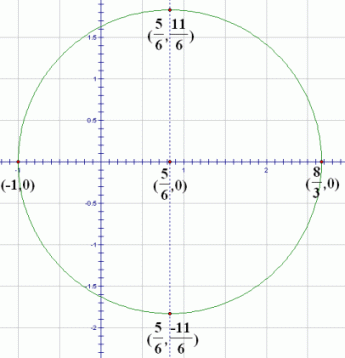﻿ Circles and the Completing the Square Technique

# CIRCLES and the ‘COMPLETING THE SQUARE’ TECHNIQUE

• PRACTICE (online exercises and printable worksheets)

Circles are useful in many Precalculus and Calculus problems (and in life).
For a thorough review, study the following two lessons.
Be sure to click-click-click the web exercises in each lesson to check your understanding!

For those of you who are short on time, however, a quick review is offered below.
It might be all that you need!

 EQUATIONS OF CIRCLES (standard form) The equation of the circle with center $\,(h,k)\,$ and radius $\,r\,$ is: $$(x-h)^2 + (y-k)^2 = r^2$$ This form of the equation is often called the standard form of a circle.For example, the circle with center $\,(2,-3)\,$ and radius $\,5\,$ might make its appearance in any of these equivalent forms:
 $(x - 2)^2 + (y - (-3))^2 = 5^2$ $(x-2)^2 + (y+3)^2 = 25$ $x^2 - 4x + 4 + y^2 + 6y + 9 = 25$ $x^2 - 4x + y^2 + 6y = 12$ $x^2 + 6y = 12 + 4x - y^2$ $2x^2 + 12y = 24 + 8x - 2y^2$

You need to be able to recognize any of these equations as a circle, and then put it in standard form and graph it.
Here are the tools you need:

RECOGNIZING CIRCLES (when they're not in standard form)
Let $\,b\,$, $\,c\,$, and $\,d\,$ be real numbers, and let $\,a\,$ be a nonzero real number.
Equations of the form $$ax^2 + ay^2+bx+cy=d$$ graph as circles.

You must have both $\,x^2\,$ and $\,y^2\,$ terms, and they must have the same coefficient,
when they are on the same side of the equation.
You are allowed (but not required) to have $\,x\,$, $\,y\,$, and constant terms.
You may not have any other term types.

It is possible to end up with a circle with radius zero,
sometimes called a ‘point’ circle, like $\,x^2+y^2 = 0\,$.
The only solution to this equation is $\,(0,0)\,$.

It is also possible to end up with an ‘imaginary’ circle, like $\,x^2 + y^2 = -1\,$.
In this case, there are no real numbers $\,x\,$ and $\,y\,$ that make the equation true.

The technique of ‘completing the square’ is needed to put a circle in standard form:

COMPLETING THE SQUARE TECHNIQUE
The process of finding the correct number to add to an expression of the form $\ x^2+bx\$ to form a perfect square trinomial is called completing the square.

The correct number to add is $\displaystyle\,{\left(\frac{b}{2}\right)}^2\,$.
That is, take the coefficient of the $\,x\,$ term, divide it by $\,2\,$, and then square the result.

Then: $$\overset{\text{start with this}}{\overbrace{x^2 + bx}} + \overset{\text{and add this number}}{\overbrace{ {\left(\frac{b}{2}\right)}^2}} = \overset{\text{to get a perfect square}}{\overbrace{ {\left(x + \frac{b}{2}\right)}^2}}$$
EXAMPLE   identifying a circle, putting in standard form, and graphing
Question:
Identify the graph of $\,3x^2 - 5x - 7 = 1 - 3y^2\,$.
If possible, graph the equation.
Solution:
There are both $\,x^2\,$ and $\,y^2\,$ term types, and they have the same coefficient when they are on the same side of the equation.
The other terms in this equation are $\,x\,$ and constant terms, which are allowable term types for a circle.
Therefore, this is the equation of a circle.

Put it in standard form as follows:

 $3x^2 - 5x - 7 = 1 - 3y^2$ original equation $3x^2 - 5x + 3y^2 = 8$ put all variable terms on the left, and constant terms on the right $\displaystyle x^2 - \frac{5}{3}x + y^2 = \frac{8}{3}$ divide both sides by $\,3\,$;the coefficient of the squared term must be $\,1\,$ to use the technique of completing the square $\displaystyle x^2 - \frac{5}{3}x + (-\frac{5}{3\cdot2})^2 + y^2 = \frac{8}{3} + \frac{25}{36}$ There is an $\,x\,$ term, so we need to complete the square to get an expression of the form $\,(x-h)^2\,$. On both sides, add $\,(\frac{-5/3}{2})^2 = (-\frac{5}{3\cdot 2})^2 = \frac{25}{36}\,$,which is the appropriate number to complete the square. $\displaystyle (x - \frac{5}{6})^2 + y^2 = \frac{121}{36}$ rename the perfect square trinomial; add fractions $\displaystyle (x-\frac{5}{6})^2 + (y-0)^2 = {(\frac{11}{6})}^2$ rename to make identification easier

Thus, this is the circle with center $\,(\frac{5}{6},0)\,$ and radius $\,\frac{11}{6}\,$.

 Once you know the center and radius of a circle, there are always four points that are easy to plot. Start at the center, and move up/down/left/right by the amount of the radius. Another way to gain confidence in your work is to check at least one of these four easy points in the original equation. Let's check the point $\,(\frac{5}{6},\frac{11}{6})\,$: $$\begin{gather} 3x^2 - 5x - 7 = 1 - 3y^2\cr 3\left(\frac{5}{6}\right)^2 - 5\left(\frac{5}{6}\right) - 7 \ \ \overset{\text{?}}{=}\ \ 1 - 3\left(\frac{11}{6}\right)^2\cr -\frac{109}{12} = -\frac{109}{12}\qquad \text{Yes!} \end{gather}$$## Using WolframAlpha To Help With Circles

For fun, jump up to WolframAlpha and type in:     properties of 3x^2 - 5x - 7 = 1 - 3y^2

How easy is that!?

Master the ideas from this section